# 随机森林过拟合问题

Random forests does not overfit. You can run as many trees as you want.

Breiman原文（在他的文章里面声明了一点，文章中的RF的模型是通过800Hz的处理器运行的），大意就是说随机森林不会过拟合，你想跑多少树就跑多少树。

y = 10 * x + noise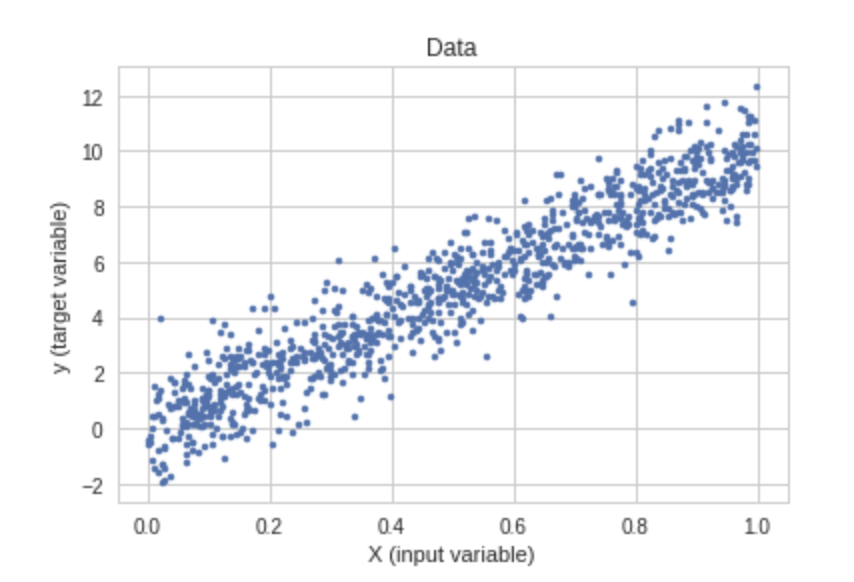data = np.random.uniform(0, 1,(1000, 1))
noise = np.random.normal(size=(1000,))
X = data[:,:1]
y = 10.0*(data[:,0]) + noise
# split to train and test
X_train, X_test, y_train, y_test = sklearn.model_selection.train_test_split(X, y, test_size=0.4, random_state=2019)

rf = RandomForestRegressor(n_estimators=50)
rf.fit(X_train, y_train)
y_train_predicted = rf.predict(X_train)
y_test_predicted_full_trees = rf.predict(X_test)
mse_train = mean_squared_error(y_train, y_train_predicted)
mse_test = mean_squared_error(y_test, y_test_predicted_full_trees)
print("RF with full trees, Train MSE: {} Test MSE: {}".format(mse_train, mse_test))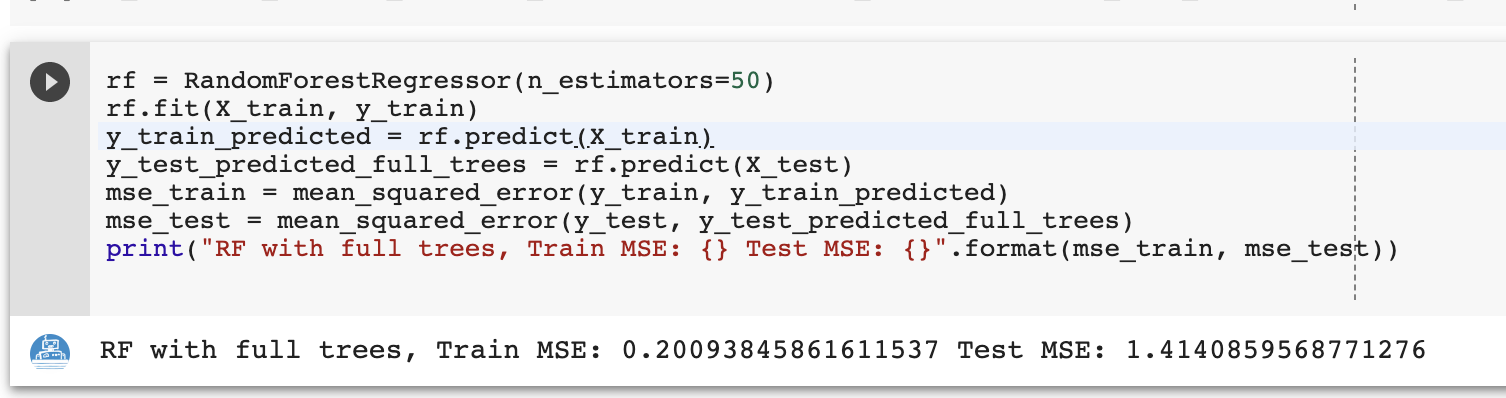rf = RandomForestRegressor(n_estimators=50, min_samples_leaf=25)
rf.fit(X_train, y_train)
y_train_predicted = rf.predict(X_train)
y_test_predicted_pruned_trees = rf.predict(X_test)
mse_train = mean_squared_error(y_train, y_train_predicted)
mse_test = mean_squared_error(y_test, y_test_predicted_pruned_trees)
print("RF with pruned trees, Train MSE: {} Test MSE: {}".format(mse_train, mse_test))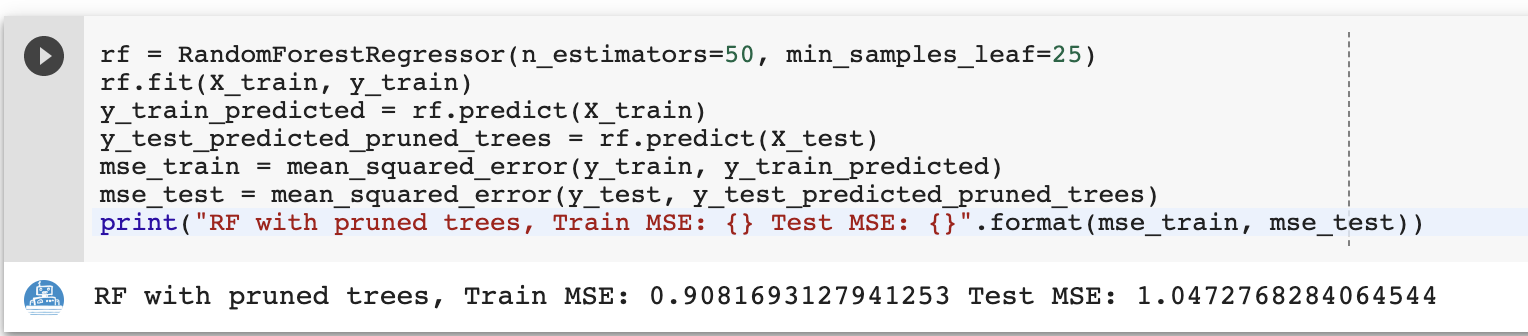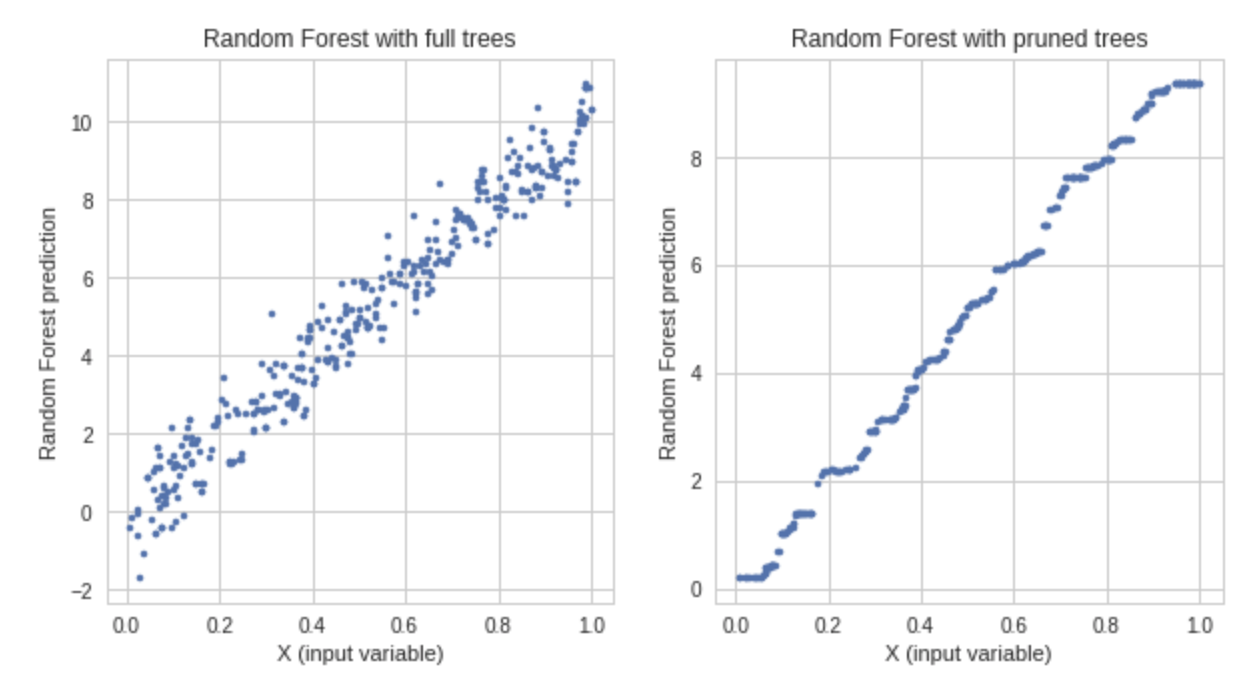rf = RandomForestRegressor(n_estimators=1)
for iter in range(50):
rf.fit(X_train, y_train)
y_train_predicted = rf.predict(X_train)
y_test_predicted = rf.predict(X_test)
mse_train = mean_squared_error(y_train, y_train_predicted)
mse_test = mean_squared_error(y_test, y_test_predicted)
print("Iteration: {} Train mse: {} Test mse: {}".format(iter, mse_train, mse_test))
rf.n_estimators += 1

plot绘制结果图：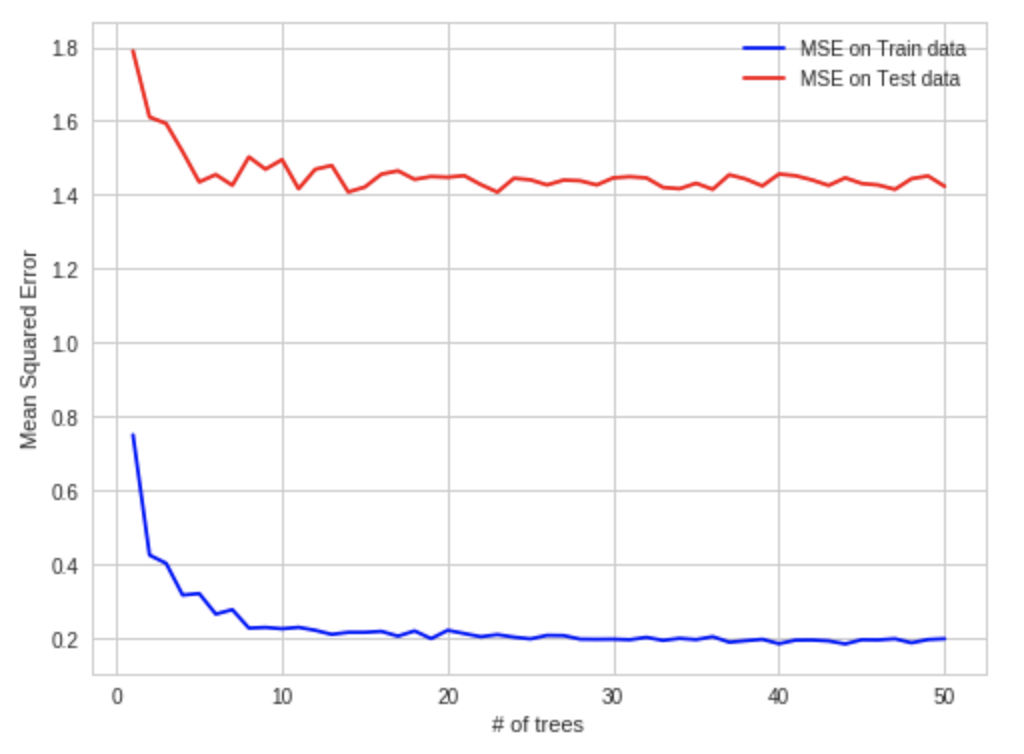• RF算法确实会过拟合。
• 当算法中加入更多的树时，随机林中的泛化误差方差将减小到零，然而，泛化的偏差并没有改变。
• 为了避免在RF中过拟合，应调整算法的超参数(hyper-parameters)，例如，叶子节点中的样本数。

04-03136108-051万+
03-211368
06-30448
04-151524
05-072804
03-082万+
06-252万+
04-253万+
03-15666
04-15647
12-291万+
01-012978
10-01536
03-172万+
03-271万+
12-061万+
10-2114万+
05-17569
07-193993